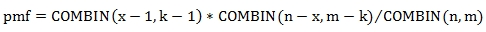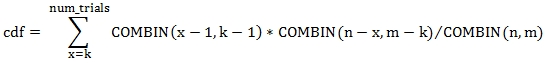# SQL Server negative hypergeometric distribution function

NEGHYPGEOM_DIST

Updated: 30 September 2015

Use NEGHYPGEOM_DIST to calculate the probability mass function or the cumulative distributive function of the negative hypergeometric distribution. The negative hypergeometric distribution models the sample size required to achieve a specified number of failures given the number of successes and the size of the population. The probability mass function can be calculated as:Where:

 x = number of trials, M = number of successes in the population, N = population size, K = number of successes to achieve with the sample, and

COMBIN calculates the binomial coefficient
The cumulative distribution function can be calculated as:Syntax
SELECT [wct].[NEGHYPGEOM_DIST](
<@num_trials, float,>
,<@num_success, float,>
,<@pop_success, float,>
,<@pop_size, float,>
,<@Cumulative, bit,>)
Arguments
@num_trials
the number of trials until @num_success have occurred. @num_trials must be of a type float or of type that intrinsically converts to float.
@num_success
the number of successes to achieve with the sample. @num_success must be of a type float or of type that intrinsically converts to float.
@pop_success
the number of successes in the population. @pop_success must be of a type float or of type that intrinsically converts to float.
@pop_size
the size of the population. @pop_size must be of a type float or of a type that intrinsically converts to float.
@Cumulative
a bit value identifying whether the probability mass function ('False') or the cumulative distribution function ('True') is to be returned. @Cumulative is of a type bit or a type that implicitly converts to bit.
Return Type
float
Remarks
·         @num_trials, @num_success, @pop_success, and @pop_size are truncated; only the integer part is used.
·         If @num_success < 0 then NULL is returned.
·         If @pop_success < 0 then NULL is returned.
·         If @num_trials = 0 then NULL is returned.
·         If @pop_size = 0 then NULL is returned.
·         If @pop_success > @pop_size then NULL is returned.
·         If @num_trials > @pop_size - @pop_success + @num_success then NULL is returned.
Examples
In an urn with 1000 balls of which 300 are white we want to calculate the probability of drawing exactly 40 white balls in 150 draws from the urn.
SELECT
wct.NEGHYPGEOM_DIST(
150          --@num_trials
,40           --@num_success
,300          --@pop_success
,1000         --@pop_size
,'False'      --@Cumulative
) as NEGHYPGEOM_DIST

This produces the following result

We can contrast this to the value returned using the binomial coefficients.
SELECT
wct.NEGHYPGEOM_DIST(
150          --@num_trials
,40           --@num_success
,300          --@pop_success
,1000         --@pop_size
,'False'      --@Cumulative
) as NEGHYPGEOM_DIST
,wct.BICO(150-1,40-1)*wct.BICO(1000-150,300-40)/wct.BICO(1000,300) as pmf

This produces the following result.

Using the same data we calculate the cumulative distribution function.
SELECT
wct.NEGHYPGEOM_DIST(
150      --@num_trials
,40       --@num_success
,300      --@pop_success
,1000     --@pop_size
,'True'   --@Cumulative
) as NEGHYPGEOM_DIST

This produces the following result.

In this example, we demonstrated the relationship between the hypergeomtric and the negative hypergeometric distibution.
SELECT
wct.NEGHYPGEOM_DIST(
150      --@num_trials
,40       --@num_success
,300      --@pop_success
,1000     --@pop_size
,'True'   --@Cumulative
) as NEGHYPGEOM_DIST
,wct.HYPGEOM_DIST(150-40,150,1000-300,1000,'True') as HYPGEOM_DIST

This produces the following result.

In this example we use the XLeratorDB SeriesInt function to show how the cdf is the sum of the pmf values from @num_success to @num_trial.

DECLARE @num_success as float = 40
DECLARE @num_trials as float = 150
DECLARE @pop_success as float = 300
DECLARE @pop_size as float = 1000

SELECT
num_trials,
pmf,
SUM(pmf) OVER (ORDER BY num_trials) as cdf
FROM (
SELECT
SeriesValue as num_trials,
wct.NEGHYPGEOM_DIST(seriesValue,@num_success,@pop_success,@pop_size,'False') as pmf
FROM
wctMath.wct.SeriesInt(@num_success,@num_trials,NULL,NULL,NULL)
)n

This produces the following result.

### SupportCopyright 2008-2021 Westclintech LLC         Privacy Policy        Terms of Service